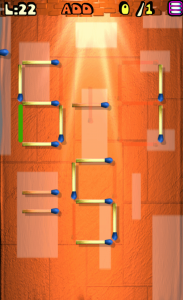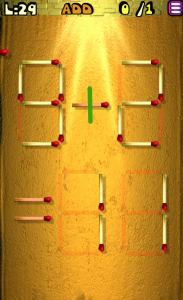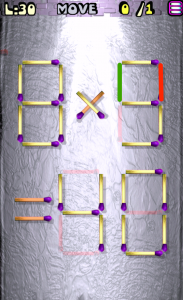# Move the Matches Puzzles Levels 21-40 Walkthrough

Walkthrough for all the solutions and answers to the game Move the Matches Puzzles by K-Lab. Get the app on the Google Play Store to play along if you do not already have it.

### Level 21Instructions: Add 4 matches to get 4 squares

### Level 22Instructions: Add 1 match to correct the equation

Solution: 6-1=5

### Level 23Instructions: Add 4 matches to get 6 squares

### Level 24Instructions: Remove 4 matches to get 5 squares, all equal

### Level 25Instructions: Move 2 matches to get 2 squares

### Level 26Instructions: Remove 1 match to correct the equation

Solution: 6+8=14

### Level 27Instructions: Remove 2 matches to correct the equation

Solution: 5+5=10

### Level 28Instructions: Remove 6 matches to get 3 triangles

### Level 29Instructions: Add 1 match to correct the equation

Solution: 9+2=11

### Level 30Instructions: Move 1 match to correct the equation

Solution: 8×5=40

### Level 31Instructions: Add 1 match to correct the equation

Solution: 8+9=17

### Level 32Instructions: Move 1 match to correct the equation

Solution: 3+6=9

### Level 33Instructions: Move 2 matches to get 3 squares

### Level 34Instructions: Add 3 matches to get 4 triangles

### Level 35Instructions: Remove 1 match to correct the equation

Solution: 7-3=4

### Level 36Instructions: Add 1 match to correct the equation

Solutions: 9-2=7

### Level 37Instructions: Add 1 match to correct the equation

Solution: 5+7=12

### Level 38Instructions: Move 2 matches to correct the equation

Solution: 8×8=64

### Level 39Instructions: Add 2 matches to get 4 triangles

### Level 40Instructions: Remove 6 matches to get 3 triangles